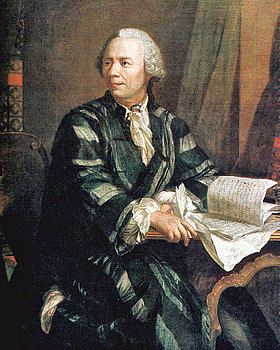# (Roughly) Daily

## Spiraling into control…The Fibonacci spiral (source)

As we remark that math really is beautiful, we might send elegantly parsimonious birthday greetings to one of Fibonacci’s spiritual descendants, a father of Pure Mathematics, Leonhard Euler; he was born on this date in 1707.  While crafting “the most remarkable formula in mathematics,” Euler made foundational contributions to number theory, graph theory, mathematical logic, and applied math; he originated many commonly-used figures of mathematical notation, and invented the concept of the “mathematical function.”  And he was no slouch in physics either, making renowned contributions in work in mechanics, fluid dynamics, optics, and astronomy.source

Written by LW

April 15, 2012 at 1:01 am

Posted in Uncategorized